# If you

If you travel to work 22 days and it takes 29.2 minutes, how many minutes will it take to travel to work and back?

Result

t =  1284.8 min

#### Solution:Leave us a comment of example and its solution (i.e. if it is still somewhat unclear...):

Showing 0 comments:Be the first to comment!## Next similar examples:

1. Conference 29 people attended a conference on behalf of their company. The conference fee was £520 per person and the company paid a total of £856 in travel costs for the 9 people. How much did the conference cost the company altogether?
2. Hours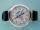How many hours is 9 days?
3. School trip6.A class went on a trip. Train ticket cost 6 euros, cable car ticket 6 euro. How many euros must pay classroom teacher for 23 students?
4. Hr to minSue biked to school in 5/12 of an hour. How many minutes did it take her to ride to school?
5. HoursThe lesson lasts 45 minutes. For the week, students have 18 lesson hours. How many are the actual hours?
6. Juice box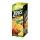In the box is 0.3 liters of juice. How many liters of juice contains 3 these boxes?
7. Seeds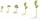Of the 72 wet seeds 7/8 germinated. How many seeds germinate?
8. The tap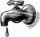For one day flows 148 l of water out of the tap. How much water will flow out for 3/4 day?
9. Decimal expansion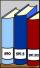Calculate: 2 . 1 + 0 . 10 + 7 . 10000 + 4 . 1000 + 6 . 100 + 0 . 100000 =
10. ProductResult of the product of the numbers 1, 2, 3, 1, 2, 0 is:
11. Expression 1What is 7+8-(5×2)+5-4+(6×(5-3)+6)-(8+10)-7+6?
12. Find the 6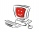Find the total cost of 10 computers at \$ 2100 each and 7 boxes of diskettes at \$12 each
13. ConcertOn a Concert were sold 150 tickets for CZK 360, 235 tickets for 240 CZK and 412 for 180 CZK. How much was the total revenues for tickets?
14. Operations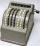Sum of the numbers 1.01 and 3.35 multiply by the difference of numbers 6.69 and 1.39.
15. Addition of Roman numbersAdded together and write as decimal number: LXVII + MLXIV
16. Roman numerals 2+Add up the number writtens in Roman numerals. Write the results as a roman numbers.
17. Roman numerals +Add up the number writtens in Roman numerals. Write the results as a decimal number.